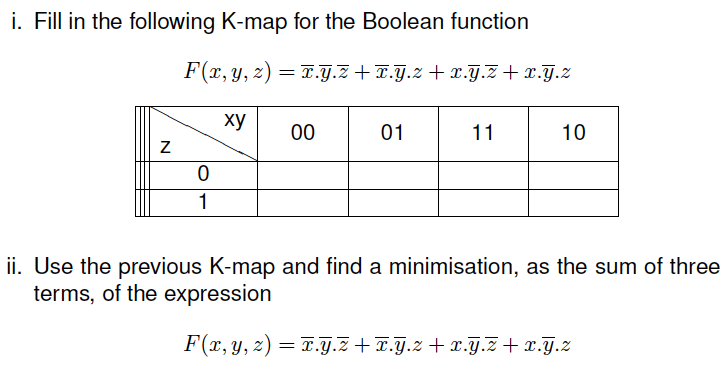# Question Solved1 Answeri. Fill in the following K-map for the Boolean function ii. Use the previous K-map and find a minimisation, as the sum of three terms, of the expression i. Fill in the following K-map for the Boolean function F(x, y, z) = r.y.z + r.y.z + r.y.z + r.y.z = ху 00 01 11 10 Z z 0 1 ii. Use the previous K-map and find a minimisation, as the sum of three terms, of the expression F(x, y, z) = 7.7.2 + r.y.z + r.y.z+x.y.zWRNDG2 The Asker · Electrical Engineering

i. Fill in the following K-map for the Boolean function
ii. Use the previous K-map and find a minimisation, as the sum of three terms, of the expressionTranscribed Image Text: i. Fill in the following K-map for the Boolean function F(x, y, z) = r.y.z + r.y.z + r.y.z + r.y.z = ху 00 01 11 10 Z z 0 1 ii. Use the previous K-map and find a minimisation, as the sum of three terms, of the expression F(x, y, z) = 7.7.2 + r.y.z + r.y.z+x.y.z
More
Transcribed Image Text: i. Fill in the following K-map for the Boolean function F(x, y, z) = r.y.z + r.y.z + r.y.z + r.y.z = ху 00 01 11 10 Z z 0 1 ii. Use the previous K-map and find a minimisation, as the sum of three terms, of the expression F(x, y, z) = 7.7.2 + r.y.z + r.y.z+x.y.z